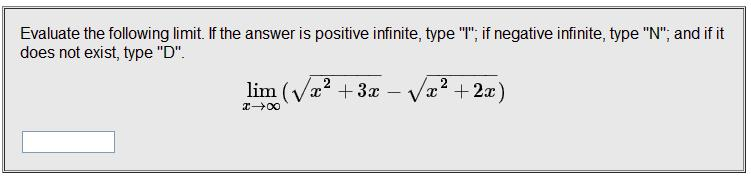# Finding Limit As X Approaches Infinite Of Square Root Function

Finding Limit As "X" Approaches Infinite Of Square Root Function

## Homework Statement## Homework Equations

None that I am aware of.

## The Attempt at a Solution

What I tried to do to solve this problem was first, multiplying the function by its conjugate, and then simplifying the numerator which yielded "5x" divided by the original conjugate. Then I factored out "x-squared" from the denominator and after taking the square root of that, I got the absolute value of "x". Since the limit approaches positive infinity, the absolute value of "x" becomes just "x" by itself. This divided out the "x's" from the numerator and the denominators. Then I simply substituted positive infinity for "x's" that remained in the square roots in the denominators and got the answer of 5/2... which is apparently wrong. Can anyone help please?

## Answers and Replies

Sourabh N

Hint 1: Factor out x2 without multiplying and dividing the conjugate.

Staff Emeritus
Homework Helper
Gold Member

## Homework Statement## Homework Equations

None that I am aware of.

## The Attempt at a Solution

What I tried to do to solve this problem was first, multiplying the function by its conjugate, and then simplifying the numerator which yielded "5x" divided by the original conjugate. Then I factored out "x-squared" from the denominator and after taking the square root of that, I got the absolute value of "x". Since the limit approaches positive infinity, the absolute value of "x" becomes just "x" by itself. This divided out the "x's" from the numerator and the denominators. Then I simply substituted positive infinity for "x's" that remained in the square roots in the denominators and got the answer of 5/2... which is apparently wrong. Can anyone help please?

What is (x2+3x)-(x2+2x) ?

It's not 5x !

Thank you Sammy, that was a silly mistake on my part.

zapz
Have you tried using ln and getting into a l'hopital's rule situation? I haven't tried it but it seems it could yield a result.

Staff Emeritus
Homework Helper
Gold Member

Have you tried using ln and getting into a l'hopital's rule situation? I haven't tried it but it seems it could yield a result.
zapz,

Try it & tell us.

(Of course it can work.) First change the expression to an appropriate form.

NasuSama

Have you tried using ln and getting into a l'hopital's rule situation? I haven't tried it but it seems it could yield a result.

It's much like the other user just said.

zapz,

(Of course it can work.) First change the expression to an appropriate form.

If you want to apply L'Hôpital Rule for this problem, then you will need to have the expression in fractional form? How? Simply multiply the top and the bottom by the conjugate of √(x² + 3x) - √(x² + 2x), which is √(x² + 3x) + √(x² + 2x). That gives us:

lim x→∞ (√(x² + 3x) - √(x² + 2x)) * (√(x² + 3x) + √(x² + 2x))/(√(x² + 3x) + √(x² + 2x))
= lim x→∞ (x² + 3x - (x² + 2x))/(√(x² + 3x) + √(x² + 2x))
= lim x→∞ (x² - x² + 3x - 2x)/(√(x² + 3x) + √(x² + 2x))
= lim x→∞ x/(√(x² + 3x) + √(x² + 2x))

If we let x = ∞, we obtain the indeterminate form, ∞/∞. For this case, we need to apply L'Hôpital Rule.

lim x→∞ d/dx [x]/(d/dx [(x² + 3x)^(½) + (x² + 2x)^(½)])
= lim x→∞ 1/(½ * (x² + 3x)^(½ - 1) * d/dx (x² + 3x) + ½ * (x² + 2x)^(½ - 1) * d/dx (x² + 2x))
= lim x→∞ 1/(½ * (x² + 3x)^(-½) * (2x + 3) + ½ * (x² + 2x)^(-½) * (2x + 2))
= lim x→∞ 1/((2x + 3)/(2√(x² + 3x)) + 2(x + 1)/(2√(x² + 2x))) [I factored out 2 out of 2x + 2]
= lim x→∞ 1/((2x + 3)/(2√(x² + 3x)) + (x + 1)/(√(x² + 2x)))

I believe you can take in from here. Find the limit of that function. Hint: This can be related to lim x→∞ Ax/(B√(x² + C)) = A/B.

Staff Emeritus
Homework Helper
Gold Member

It's much like the other user just said.

If you want to apply L'Hôpital Rule for this problem, then you will need to have the expression in fractional form? How? Simply multiply the top and the bottom by the conjugate of √(x² + 3x) - √(x² + 2x), which is √(x² + 3x) + √(x² + 2x). That gives us:
If you multiply by the conjugate over the conjugate , then simplify, and factor x (x2 under the radicals) from the radicals, you don't need L'Hôpital's rule .

However:
To use L'Hôpital's rule, start with [BSourabh N][/B]'s suggestion in post#2.

$\displaystyle \sqrt{x^2+3x}-\sqrt{x^3+2x}$

$\displaystyle =x\sqrt{1+\frac{3}{x}}-x\sqrt{1+\frac{2}{x}}$

$\displaystyle =\frac{\sqrt{1+3/x}-\sqrt{1+2/x}}{1/x}$
This is of the form 0/0 as x → ∞ .

Apply L'Hôpital's rule to the limit of this expression.